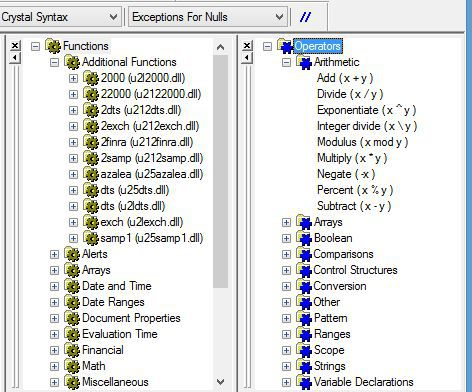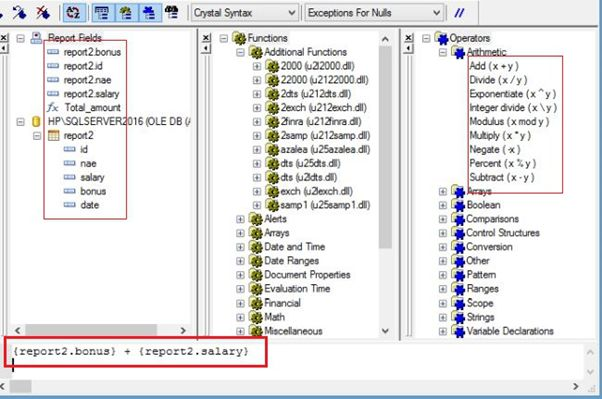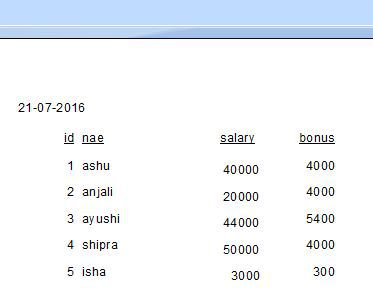# Applying Calculations

We know that the formulas are applied to solve the big calculation in any field. Thus, in Crystal Report, we use the formulas in ‘Formula Workshop’ to making the calculation, easy and fast. Crystal Report allows many operators to be used in the formula like arithmetic, comparison etc.When we write the formula, pick the object field from ‘Report Field’ and then pick the 'Operator', which we want to allow in our formula.Now, save the formula with ‘Save and Close’ option, go to Design page of the record and drag the formula name on the report. After this, we can see the result on the report page.List of some common operators are as follows,